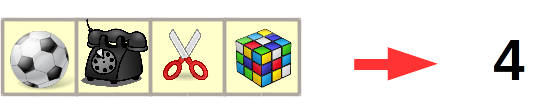# Programming-Idioms# Idiom #117 Get list size

Set n to the number of elements of the list x.``def n = x.size()``
``````with Ada.Containers.Vectors;
``N : Count_Type := X.Length;``
``````int n;
int x;
n = sizeof(x)/sizeof(*x);``````
``size_t n = sizeof(x)/sizeof(*x);``
``(def n (count x))``
``size_t n = x.size();``
``using System.Collections.Generic;``
``int n = x.Count;``
``int n = x.Length;``
``auto n = x.length;``
``import std.range.primitives;``
``n = walkLength(x);``
``int n = x.length;``
``n = Enum.count(x)``
``N = length(X).``
``n = size(x)``
``n := len(x)``
``let n = length x``
``var n = x.length;``
``int n = x.size();``
``int n = x.length;``
``val n = x.size``
``(setf n (length x))``
``local n = #x``
``@import Foundation;``
``NSUInteger n=x.count;``
``\$n = count(\$x);``
``n := length(x);``
``\$n = scalar @x;``
``````my \$N = @x;
``````
``n = len(x)``
``n = x.length``
``````let n = x.len();

``````
``val n = x.size``
``(define n (length x))``
``n := x size.``
``Dim n As Integer = x.Count``
``n = UBound(x) + 1``

programming-idioms.org